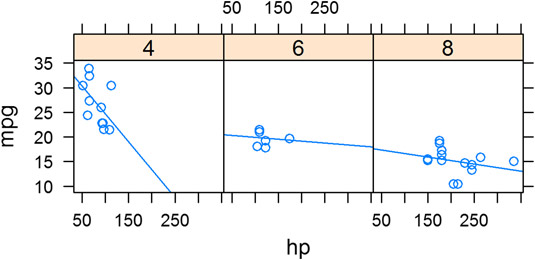##### R For DummiesYou can create trend lines or regression lines through data. These can be useful when using lattice plots in R. When you tell lattice to calculate a line of best fit, it does so for each panel in the plot. This is straightforward using xyplot(), because it’s as simple as adding a type argument.

In particular, you want to specify that the type is both points (type = p) and regression (type = r). You can combine different types with the c() function, like this:

```> xyplot(mpg ~ hp | cyl, data = transform.mtcars,
+    type = c("p", "r"))```

Your graphic should look like this.xyplot with regression lines added." width="535"/>
Lattice xyplot with regression lines added.

Strictly speaking, type is not an argument to xyplot(), but an argument to panel.xyplot(). You can control the panels of lattice graphics with a panel function. The function xyplot() calls this panel function internally, using the type argument you specified. The default panel function for xyplot() is panel.xyplot().

Similarly, the panel function for barchart() is panel.barchart(). The panel function allows you to take fine control over many aspects of your chart. You can find out more in the excellent Help for these functions — for example, by typing ?panel.xyplot into your R console.